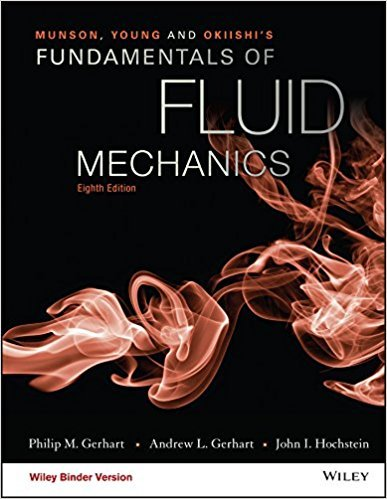×
×

# Consider the possibility of using two rotating cylinders toreplace the conventionalISBN: 9781119080701 456

## Solution for problem 6.65 Chapter 6.6

Fundamentals of Fluid Mechanics | 8th Edition

• Textbook Solutions
• 2901 Step-by-step solutions solved by professors and subject experts
• Get 24/7 help from StudySoup virtual teaching assistantsFundamentals of Fluid Mechanics | 8th Edition

4 5 1 282 Reviews
22
2
Problem 6.65

Consider the possibility of using two rotating cylinders toreplace the conventional wings on an airplane for lift. Consider anairplane flying at 700 km/hr through the Standard Atmosphere at10,000 m. Each wing cylinder has a 3.0-m radius. The surfacevelocity of each cylinder is 28 km/hr. Find the length of eachwing to develop a lift of 1550 kN. Assume potential flow andneglect end effects.

Step-by-Step Solution:
Step 1 of 3

Naming ions -Cations (metals) keep the name of the metal -Anions (nonmetals) end in -ide -transition metals get a Roman Numeral to specify their charge Sn —> tin (II) 4+ transition metals Sn —> tin (V) Ag —> Silver (I) 2+ Ba —> barium...

Step 2 of 3

Step 3 of 3

##### ISBN: 9781119080701

Since the solution to 6.65 from 6.6 chapter was answered, more than 213 students have viewed the full step-by-step answer. This textbook survival guide was created for the textbook: Fundamentals of Fluid Mechanics, edition: 8. The answer to “Consider the possibility of using two rotating cylinders toreplace the conventional wings on an airplane for lift. Consider anairplane flying at 700 km/hr through the Standard Atmosphere at10,000 m. Each wing cylinder has a 3.0-m radius. The surfacevelocity of each cylinder is 28 km/hr. Find the length of eachwing to develop a lift of 1550 kN. Assume potential flow andneglect end effects.” is broken down into a number of easy to follow steps, and 62 words. This full solution covers the following key subjects: . This expansive textbook survival guide covers 112 chapters, and 1357 solutions. Fundamentals of Fluid Mechanics was written by and is associated to the ISBN: 9781119080701. The full step-by-step solution to problem: 6.65 from chapter: 6.6 was answered by , our top Science solution expert on 03/16/18, 03:21PM.

Unlock Textbook Solution